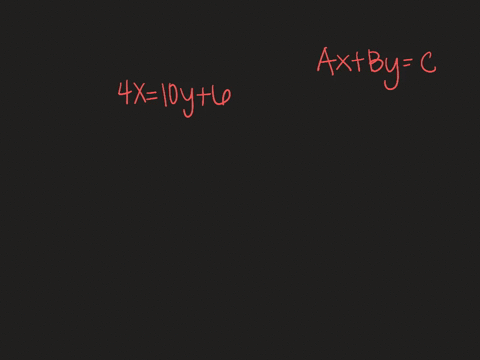Enroll in one of our FREE online STEM summer camps. Space is limited so join now!View Summer Courses### Write each equation in standard form. Identify A,…

00:43University of North Texas

Need more help? Fill out this quick form to get professional live tutoring.

Get live tutoring
Problem 5

Write each equation in standard form. Identify A, B, and C.
$y=3 x-5$

$A=3, B=-1, C=5$

## Discussion

You must be signed in to discuss.

## Video Transcript

It wants us to write this equation in standard form and then identify a B and C so standard for miss a X plus y equal. See? So I want to subtract three X on both sides. So I have negative three X plus y equals negative five. So a needs to be positive. So we're gonna divide off one on every number. So I get three x minus y equals five. All that does This changes all of our signs. So three is a be is a negative one because there is a visible one here and see is five.